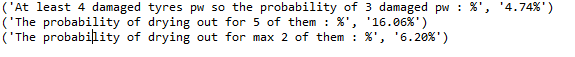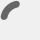### self in Python

Category : Code, Python

Only Turkish version is available.

### Playing with Google Chromecast

Category : chromecast, Code, Python

Only Turkish version is available!

### Poisson Probability

Category : Code, General, Python

Code :

```import math

# Questions are translated from https://www.youtube.com/watch?v=3-539wQLCaQ

#Example 1
#A tire company producing tires during 5 days in a week. 4 of tires averagely
#are failed in a weekly based production. What is the probability
#of at least 3 failed tires production in a day in the company,

def poisson_probability(actual, mean):
# naive:   math.exp(-mean) * mean**actual / factorial(actual)

# iterative, to keep the components from getting too large or small:
p = math.exp(-mean)
for i in xrange(actual):
p *= mean
p /= i+1
return p

def example1():
mean = 4./5
prob = poisson_probability(0,mean) + poisson_probability(1,mean) + poisson_probability(2,mean)
prob = 1 - prob
percent_str = '{:.2%}'.format(prob)
print("EX1 : At least 4 damaged tyres pw so the probability of 3 damaged pw : %", percent_str)

def example2():
mean = 3*2    #mean for 2 decares
prob = poisson_probability(5,mean)
percent_str = '{:.2%}'.format(prob)
print("EX2(a) : The probability of drying out for 5 of them : %", percent_str)

prob = poisson_probability(0,mean) + poisson_probability(1,mean) + poisson_probability(2,mean)
percent_str = '{:.2%}'.format(prob)
print("EX2(b) :The probability of drying out for max 2 of them : %", percent_str)

if __name__ == '__main__':
example1()
example2()
pass```

Output :

(‘At least 4 damaged tyres pw so the probability of 3 damaged pw : %’, ‘4.74%’)
(‘The probability of drying out for 5 of them : %’, ‘16.06%’)
(‘The probability of drying out for max 2 of them : %’, ‘6.20%’)error: Content is protected !!